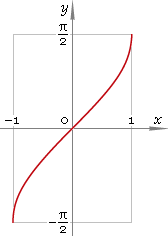The Art of Interface

# arcsin — trigonometric arc sine function

Category. Mathematics.

Abstract. Trigonometric arc sine: definition, plot, properties, identities and table of values for some arguments.

## 1. Definition

Arc sine is inverse of the sine function.

## 2. Plot

Arc sine is monotone antisymmetric function defined in the range [−1, 1]. Its plot is depicted below in fig. 1.Fig. 1. Plot of the arc sine function y = arcsinx.

Function codomain is limited to the range [−π/2, π/2].

## 3. Identities

Complementary angle:

arcsinx + arccosx = π/2

and as consequence:

arcsin cos φ = π/2 − φ

Negative argument:

arcsin(−x) = −arcsinx

Reciprocal argument:

arcsin(1/x) = arccscx

Sum and difference:

arcsinx + arcsiny = arcsin[x√(1 − y2) + y√(1 − x2)]
arcsinx − arcsiny = arcsin[x√(1 − y2) − y√(1 − x2)]

Some argument values:

Argument xValue arcsinx
00
(√6 − √2) /4π/12
(√5 − 1) /4π/10
√(2 − √2) /2π/8
1 /2π/6
√(10 - 2√5) /4π/5
1 /√2π/4
(√5 + 1) /43π/10
√3 /2π/3
√(2 + √2) /23π/8
√(10 + 2√5) /42π/5
(√6 + √2) /45π/12
1π/2
Table 1. Arc sine for some argument values.

## 4. Support

Trigonometric arc sine function arsin of the real argument is supported by free version of the Librow calculator.

Trigonometric arc sine function arsin of the complex argument is supported by professional version of the Librow calculator.

## 5. How to use

To calculate arc sine of the number:

``arcsin(-1);``

To calculate arc sine of the current result:

``arcsin(rslt);``

To calculate arc sine of the number x in memory:

``arcsin(mem[x]);``